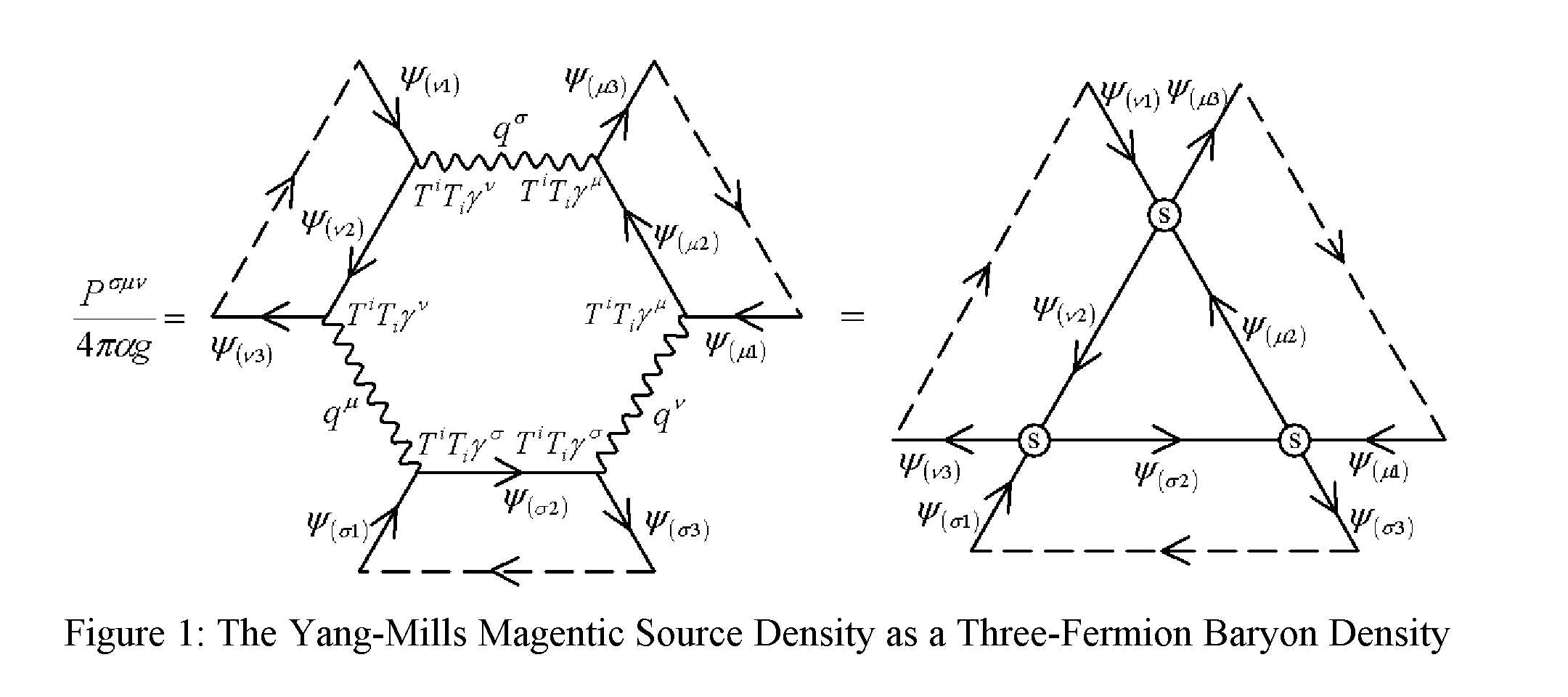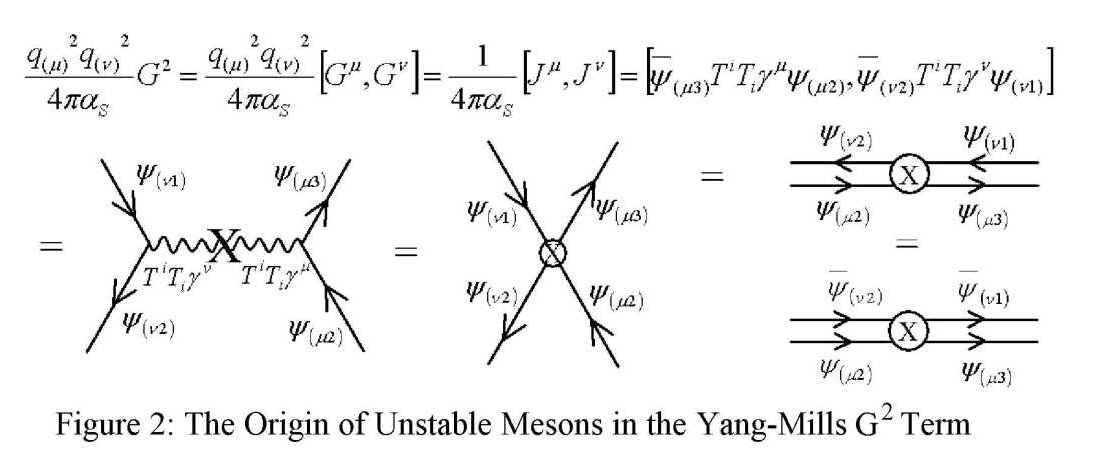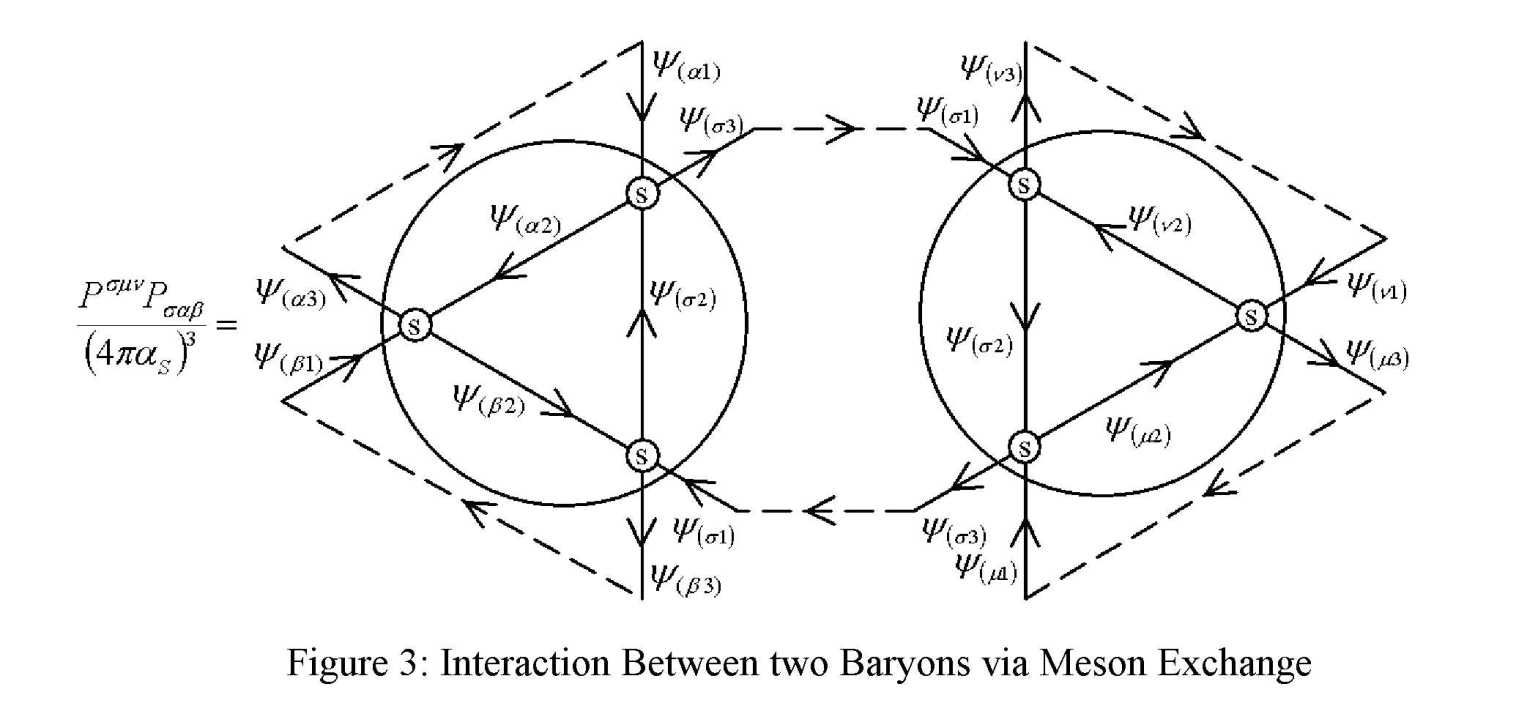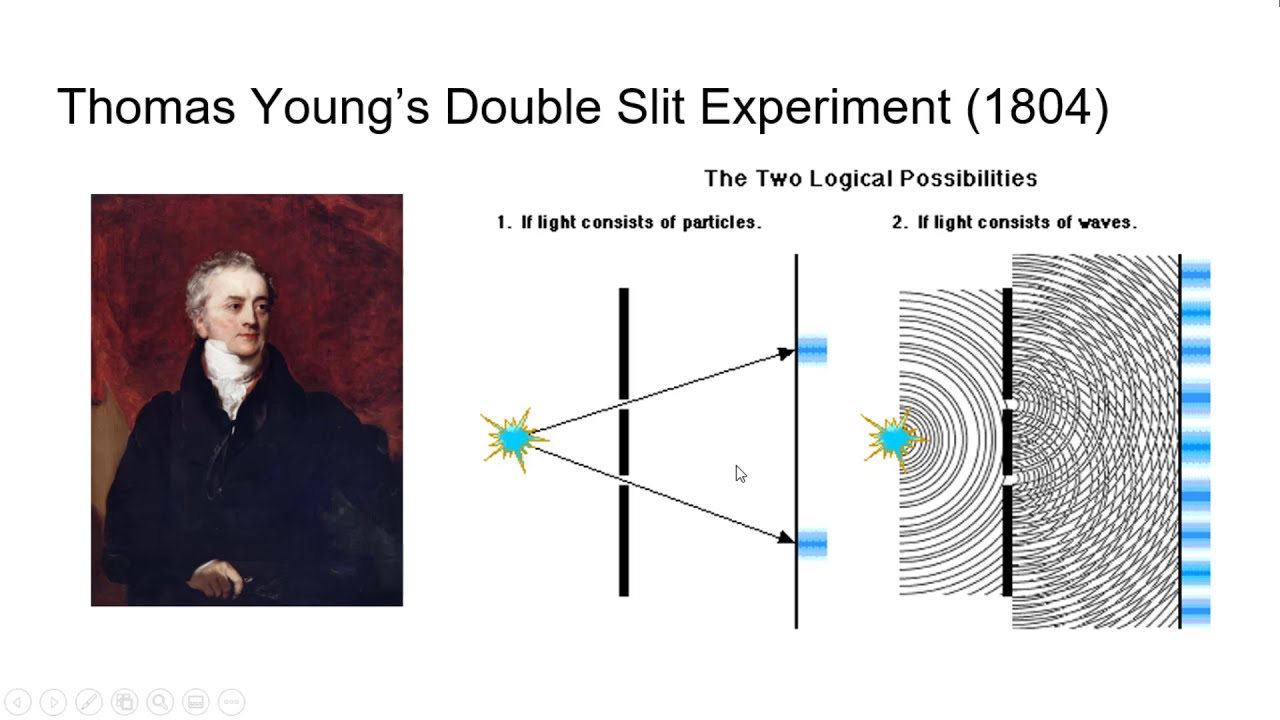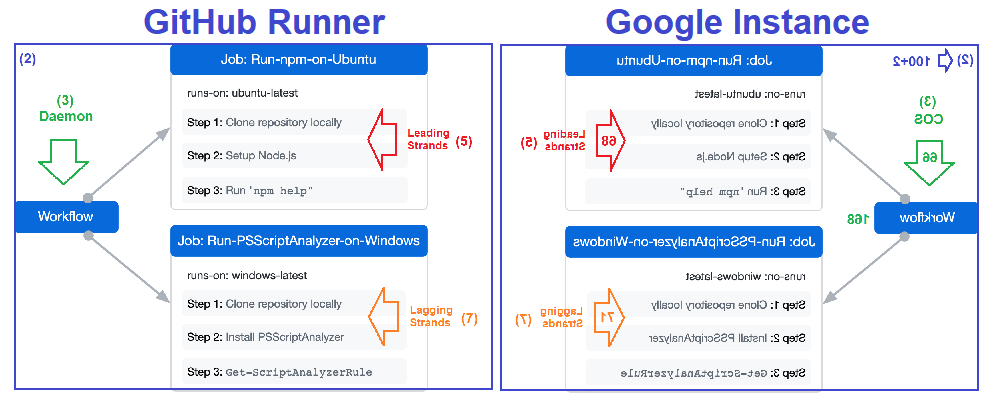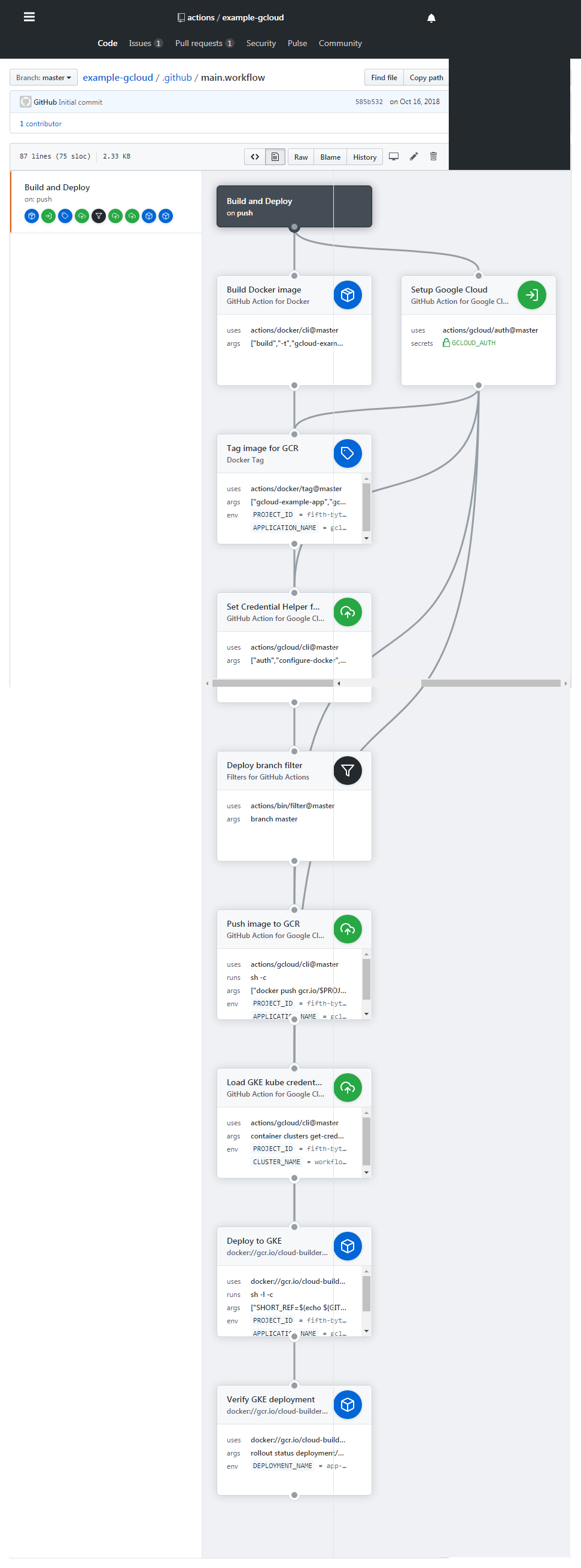## Mass vs Gap (Δ)

This section will be the last one of our presentation of 18th prime identity. Here we are going to explain one more item that is still open or undiscussed which is about what the 77 objects are going to do within the 19 vs 18 Scenario.

15 + 35 + 28 = 15 + 63 = 78 = 77 + 1

``````---+-----+-----
1 | 1   | 15
---+-----+-----
2 | 16  | 25
---+-----+-----
3 | 26  | 50
---+-----+-----
4 | 51  | 84
---+-----+-----
5 | 85  | 99
---+-----+-----
``````
``````\$True Prime Pairs:
(5,7), (11,13), (17,19)

layer|  i  |   f
-----+-----+---------
|  1  | 5
1  +-----+
|  2  | 7
-----+-----+---  } 36 » 6®
|  3  | 11
2  +-----+
|  4  | 13
-----+-----+---------
|  5  | 17
3  +-----+     } 36 » 6®
|  6  | 19
-----+-----+---------
``````

In many ways, a black hole acts like an ideal black body, as it reflects no light. Here is an animated simulation of a Schwarzschild black hole with a galaxy passing behind. Around the time of alignment, extreme gravitational lensing of the galaxy is observed.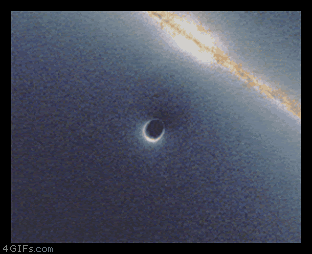``````                largest part=21 → 11+13+12=36 →  MEC30
↓                      |
---+-----+-----+-----+-----+                   ↓
1 | 19  | 1   | 20  | 21  |-------------------|-----
---+-----+-----+-----+-----+                   ↓     |
2 | 18  | 21  | 39  | 60  |-------------------      |
---+-----+-----+-----+-----+                   |     |
3 |{63} | 40  | 103 | 143 |-------------      |     |
---+-----+-----+-----+-----+             |     |     |
4 | 37  | 104 | 141 | 245 |-------      |     |     |
---+-----+-----+-----+-----+       |     |     |     |
5 | 10* | 142 | 152 | 294 |- 11** | 13  | 12  | 12  | 18
---+-----+-----+-----+-----+       |     |     |     |
6 | 24  | 153 | 177 | 332 |-------      |     |     |
---+-----+-----+-----+-----+             |     |     |
7 | 75  | 178 | 253 | 431 |-------------      |     |
---+-----+-----+-----+-----+                   |     |
8 | 30  | 254 | 284 | 538 |-------------------      |
---+-----+-----+-----+-----+                   ↓     |
9 | 1   | 285 | 286 | 571 |-------------------|-----
===+=====+=====+=====+=====+                   ↓
45 | 277 |                      ← 11+13+12=36 ←  MEC30
---+-----+                                     |
↑
Note:
10* stands as the central rank
11** stands as the central parts
``````

According to the observations made by NASA, Astronomers have uncovered TON 618 as the record breaking supermassive black hole, weighing 66 trillion and brilliantly as 140 trillion times that of the Sun, making it one of the brightest object in the Universe.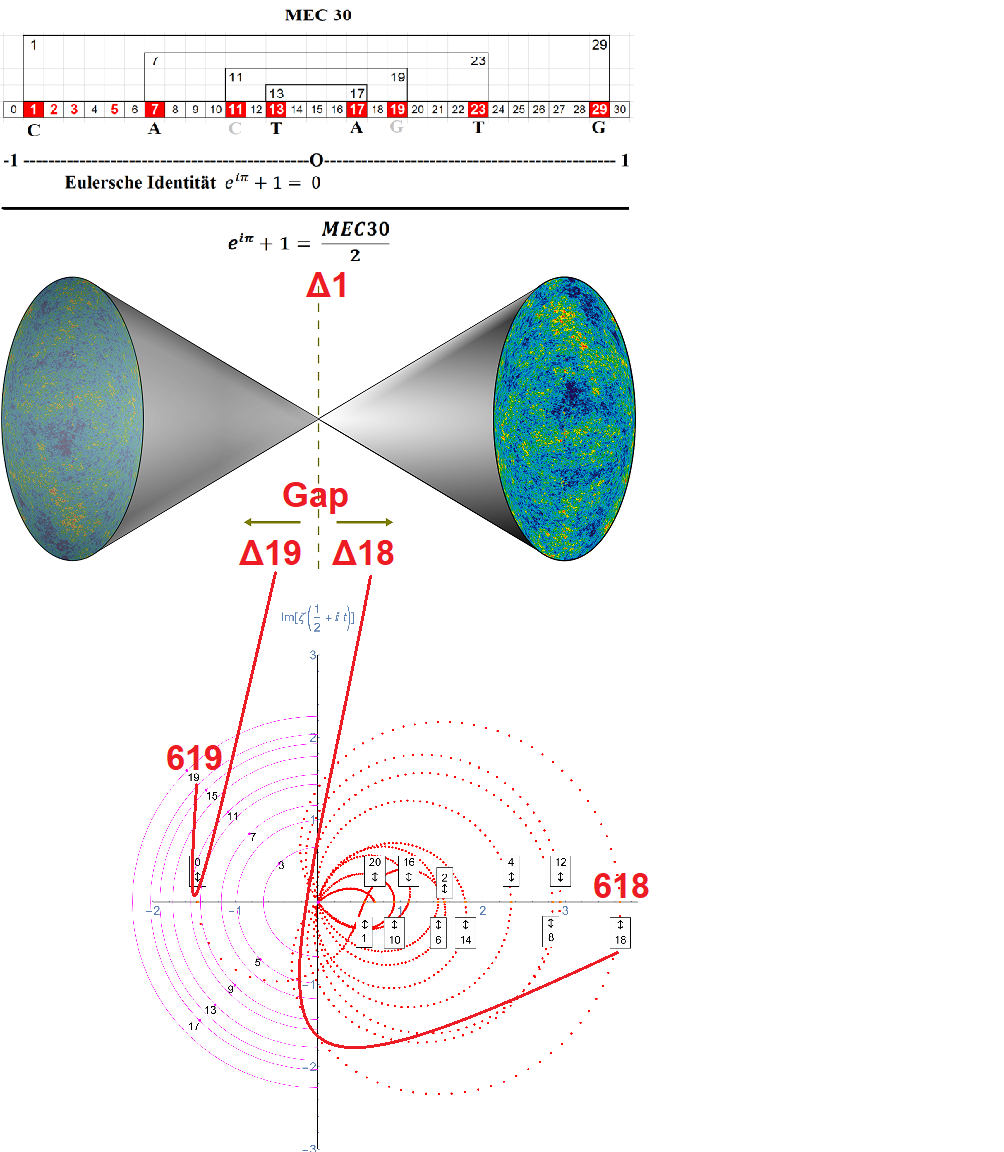If the statement that it is indeed located at the center of our universe then the said black hole would behave as the exchange position between twin (2) universes. This would for sure strengthen the syntax algorithm of our implementation.

7 x 11 = 77 = 99 - 22 = 11 x (9 -2)

``````  #8  |------- 5® --------|------------ 7® --------------|
| 1 |-------------- 77 = 4² + 5² + 6² -------------|
------+---|---+---+---+---+---+---+---+---+----+----+----+
repo |{1}|{2}| 3 | 4 | 5 | 6 | 7 | 8 | 9 | 10 | 11 |{12}| 1,77
------+---|---+---+---+---+---+---+---+---+----+----+----+
user | 7 | - | - | - | - | 7 | 8 | - | - |  8 |  8 |  3 |
------+---|---+---+---+---+---+---+---+---+----+----+----+ 7,78
main | - | 9 | 7 | 9 | 6 | - | - | 8 | 5 |  - |  - |  - |
------+---|---+---+---+---+---+---+---+---+----+----+----+
Δ | Δ             |                      Δ  |   Δ
Φ17|Φ29            |                    96-99|  100 - 123 ({24})
|--- A,T,G,C ---|                         |  └── 100 - 103 (4x) » 100
Δ    2x2 = 4x   |-------  2x3 = 6x -------|  └── 104 - 109 (6x) » 30
{98}                                       |  └── 110 - 123 (14x)» 70

``````
``````Direction:
- The initial of 168 & 329 brings the 102 as 100+2 to π(π(10000))-1=200 or 100 x 2.
- Then the 289 lets this 100x2 to 100² so it brings 100 to 10000 by the power of 2.
- At the last it will be separated by the scheme of 168 to 102 goes back 100 and 2.

Conclution:
- All of the other primes than 2 is 1 less than the number n times the number of 2.
- Those Mersenne primes is generated as 1 less than the power n of the number of 2.
- Thus they will conseqently be carried out by the same scheme of this number of 2.
``````

Perceptually, everything is separate and finite. But actually, everything is connected and infinite. It is this infinite connection, despite our limited finite perceptions, that makes us one with the cosmos.

## Primes Platform

Each result goes to the 9th object of prime 67 which is 19th prime. This mass gap of (Δ > 0) is actually the quantum way of our algorithm which is discused in details by the 19th prime identity.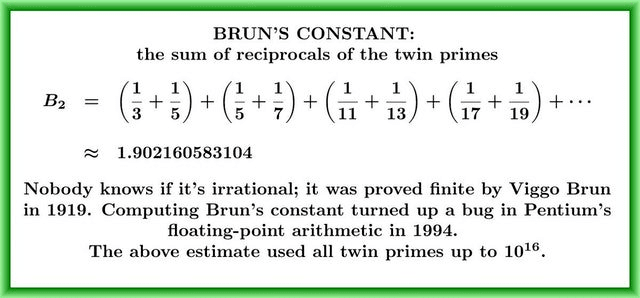So when the cycle has passed the 10th object then the 43 objects will be laid by 9 collumns and slightly forming bilateral 9 sum which facilitate them to finaly generate 1000 primes.

1 instance + 7 blocks + 29 flats + 77 rooms = 114 objects

``````True Prime Pairs:
(5,7), (11,13), (17,19)

-----+-----+-----+-----+-----+     -----------------------------------------------
{786}| 1,2 |  2  | 2,3 | 3,4 | {19}                                          |
-----+-----+-----+-----+-----+                                               |
{86}|  4  | 4,5 | 5,6 |{6,7}| 17                                        Base Zone
+-----+-----+-----+-----+                                               |
{78}|{7,8}| 8,9 | 12 (M dan F) ----> Δ                                      |
+-----+-----+-----+                                               -----------
{67}| 9,11|11,12|12,14| 11 <----------- Mid Zone                            |
----+-----+-----+-----+-----+                                               |
{6}|15,16|17,18|18,20|21,22| 19                                      Mirror Zone
+-----+-----+-----+-----+                                               |
{8}|23,25|25,27|27,29| 18                                                  |
+-----+-----+-----+-----+-----+-----+-----+-----+-------+         -----------
{7}|29,33|33,36|36,39|39,41|41,45|46,51|51,57|58,66|{67,77}| 43 (C1 dan C2)<---Δ
-----+-----+-----+-----+-----+-----+-----+-----+-----+-------+         -----------
|  1     2     3  |   4     5     6 |   7     8      9  |
|------ 29' ------|--------------- 139' ----------------|
|------ 102¨ -----|---------------  66¨ ----------------|
``````

When recombination is occur then the prime 13 is forced to → 12 where the impact (Δ1) goes to 18+13+12=43 on the last 7th row forming the Primes Platform. Thus we got 109 objects including for the 7 rows back to the original stage.

``````  Tabulate Prime by Power of 10
loop(10) = π(10)-π(1) = 4-0 = 4
loop(100) = π(100)-π(10)-1th = 25-4-2 = 19
loop(1000) = π(1000) - π(100) - 10th = 168-25-29 = 114

-----------------------+----+----+----+----+----+----+----+----+----+-----
True Prime Pairs Δ    |  1 |  2 |  3 |  4 |  5 |  6 |  7 |  8 |  9 | Sum
=======================+====+====+====+====+====+====+====+====+====+=====
19 → π(10)            |  2 |  3 |  5 |  7 |  - |  - |  - |  - |  - | 4th  4 x Root
-----------------------+----+----+----+----+----+----+----+----+----+-----
17 → π(20)            | 11 | 13 | 17 | 19 |  - |  - |  - |  - |  - | 8th  4 x Twin
-----------------------+----+----+----+----+----+----+----+----+----+-----
13 → π(30) → 12 (Δ1)  | 23 | 29 |  - |  - |  - |  - |  - |  - |  - |10th
=======================+====+====+====+====+====+====+====+====+====+===== 1st Twin
11 → π(42)            | 31 | 37 | 41 |  - |  - |  - |  - |  - |  - |13th
-----------------------+----+----+----+----+----+----+----+----+----+----- 2nd Twin
7 → π(60) → 19 (Δ12) | 43 | 47 | 53 | 59 |  - |  - |  - |  - |  - |17th
-----------------------+----+----+----+----+----+----+----+----+----+----- 3rd Twin
5 → π(72) → 18 (Δ13) | 61 | 67 | 71 |  - |  - |  - |  - |  - |  - |20th
=======================+====+====+====+====+====+====+====+====+====+===== 4th Twin
3,2 → 18+13+12 → 43  | 73 | 79 | 83 | 89 | 97 | 101| 103| 107| 109|29th
=======================+====+====+====+====+====+====+====+====+====+=====
Δ                                                            Δ
12+13+(18+18)+13+12   ← 36th-Δ1=151-1=150=100+2x(13+12)   ←   30th = 113 = 114-1

Sequence:
By the next layer the 89² will become 89 and 5 become 5² or 25.
This 89 and 25 are in the same layer with total of 114 or prime 619
So sequence from the first prime is 1,4,7,10,29,68,89,114,139,168,329,618.
``````

[(6 + 6) x 6] + [6 + (6 x 6)] = 72 + 42 = 71 + 42 + 1 = 114 objects

``````The Prime Recycling ζ(s):
(2,3), (29,89), (36,68), (72,42), (100,50), (2,3), (29,89), ...**infinity**
----------------------+-----+-----+-----+                                    ---
7 --------- 1,2:1|   1 |  30 |  40 | 71 (2,3) ‹-------------@----        |
|                +-----+-----+-----+-----+                        |      |
|  8 ‹------  3:2|   1 |  30 |  40 |  90 | 161 (7) ‹---           |      5¨  encapsulation
|  |             +-----+-----+-----+-----+             |          |      |
|  |  6 ‹-- 4,6:3|   1 |  30 | 200 | 231 (10,11,12) ‹--|---       |      |
|  |  |          +-----+-----+-----+-----+             |   |      |     ---
--|--|-----» 7:4|   1 |  30 |  40 | 200 | 271 (13) --›    | {5®} |      |
|  |          +-----+-----+-----+-----+                 |      |      |
--|---› 8,9:5|   1 |  30 | 200 | 231 (14,15) ---------›       |      7¨  abstraction
289        |          +-----+-----+-----+-----+-----+                  |      |
|          ----› 10:6|  20 |   5 |  10 |  70 |  90 | 195 (19) --› Φ   | {6®} |
--------------------+-----+-----+-----+-----+-----+                  |     ---
67 --------› 11:7|   5 |   9 |  14 (20) --------› ¤               |      |
|                +-----+-----+-----+                              |      |
|  78 ‹----- 12:8|   9 |  60 |  40 | 109 (26) «------------       |     11¨  polymorphism
|  |             +-----+-----+-----+                       |      |      |
|  |  86‹--- 13:9|   9 |  60 |  69 (27) «-- Δ19 (Rep Fork) | {2®} |      |
|  |  |          +-----+-----+-----+                       |      |     ---
|  |   ---› 14:10|   9 |  60 |  40 | 109 (28) -------------       |      |
|  |             +-----+-----+-----+                              |      |
|   ---› 15,18:11|   1 |  30 |  40 | 71 (29,30,31,32) ----------        13¨  inheritance
329  |                +-----+-----+-----+                                     |
|   ‹--------- 19:12|  10 |  60 | {70} (36) ‹--------------------- Φ        |
-------------------+-----+-----+                                          ---
786 ‹------- 20:13|  90 |  90 (38) ‹-------------- ¤                      |
|                +-----+-----+                                           |
| 618 ‹- 21,22:14|   8 |  40 |  48 (40,41) ‹----------------------      17¨  class
|  |             +-----+-----+-----+-----+-----+                  |      |
|  | 594 ‹- 23:15|   8 |  40 |  70 |  60 | 100 | 278 (42) «--     |{6'®} |
|  |  |          +-----+-----+-----+-----+-----+             |    |     ---
--|--|-»24,27:16|   8 |  40 |  48 (43,44,45,46) ------------|----       |
|  |          +-----+-----+                               |           |
--|---› 28:17| 100 | {100} (50) ------------------------»           19¨  object
168        |          +-----+                                                 |
|         102 -› 29:18| 50  | 50(68) ---------> Δ18                           |
----------------------+-----+                                                ---
``````

The above is observed following the W0 (assumptions of relativistic quantum mechanics) for the Existence and Mass Gap which transform under the homogeneous group as a four-vector and has a mass gap Δ > 0.

Yang–Mills Existence and Mass Gap. Prove that for any compact simple gauge group G, a non-trivial quantum Yang–Mills theory exists on R^4 and has a mass gap Δ > 0 (Wikipedia).